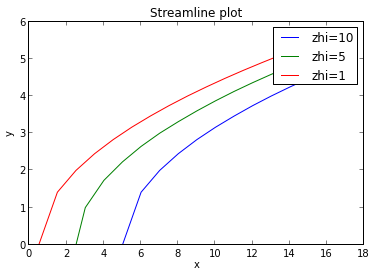## Chapter 3: Fluid Kinematics¶

### Example 3.1 Page no 117¶

In :
# Dimension of flow field ; velocity components at (1,2) ; magnitude and direction of velocity

from math import *

# Given

# V = 4*Xi-4Yj

x=1 # x co-ordinate

y=2 # y co-ordinate

# Solution

print "(a) u = 4*X; v = -4*Y "

u = 4*x

v=- 4*y

print "(b) u=",round(u,0),"m/s and v=",round(v,0),"m/s"

R =sqrt(u**2+v**2)

ang = atan(v/u)*180/pi

print "(c) Magnitude of velocity =",round(R,2),"m/s and angle of resultant velocity = ",round(ang,1),"deg"

(a) u = 4*X; v = -4*Y
(b) u= 4.0 m/s and v= -8.0 m/s
(c) Magnitude of velocity = 8.94 m/s and angle of resultant velocity =  -63.4 deg


### Example 3.2 Page no 119¶

In :
# Discharge and mass flow rate

from math import *

from __future__ import division

# Given

d = 0.3                  # diameter of pipe in m

v = 15                   # velocity in m/s

rho = 997.1              # density in kg/m**3

A = pi*d**2/4

# Solution

Q=A*v

print "(a) Discharge =",round(Q,2),"m**3/s"

mdot = rho*Q

print "(b) Mass flow rate = ",round(mdot,2),"kg/s"

(a) Discharge = 1.06 m**3/s
(b) Mass flow rate =  1057.21 kg/s


### Example 3.3 Page no 120¶

In :
# Mean Velocity

from math import *

from __future__ import division

from scipy import integrate

# Given

Vo = 10                  # velocity in m/s

r1 = 0

ro = 0.1                 # radius in m

N = 1

# Solution

R = lambda r: (10*r-1000*r**3)

Q =  R1*2*pi

A = pi*(0.1)**2

V = Q/A

print "Mean velocity of the flow =",round(V,0),"m/s"

Mean velocity of the flow = 5.0 m/s


### Example 3.4 Page no 126¶

In :
# Sketch the stream lines in the first quadrant

import matplotlib.pyplot as plt

from math import *

from scipy import integrate

import numpy as np

from sympy import *

#init_printing(use_unicode=False, warp_line=False, no_global=True)

# Given

# V = 4*y(m)i+2(m)j

x = Symbol('x')
U = integrate(2,x)

#print u

y = Symbol('y')

V = integrate(-4*y,y)

#print V

Zhi = U + V

print Zhi           # for x and y =0 we get C = 0

X = [5,6,7,8,9,10,11,12,13,14,15,16,17]
Y = [0,1.414,2,2.449,2.828,3.16,3.46,3.741,4,4.242,4.472,4.69,4.898]

b1=plt.plot(X,Y)

X1 = [2.5,3,4,5,6,7,8,9,10,11,12,13,14,15]
Y1 = [0,1,1.732,2.23,2.645,3,3.31,3.60,3.87,4.123,4.35889,4.5825,4.795,5]

b2=plt.plot(X1,Y1)

X2 = [0.5,1.5,2.5,3.5,4.5,5.5,6.5,7.5,8.5,9.5,10.5,11.5,12.5,13.5,14.5,15.5]
Y2 = [0,1.414,2,2.449,2.828,3.162,3.462,3.741,4,4.242,4.472,4.69,4.898,5.099,5.29,5.4772]

b3=plt.plot(X2,Y2)

plt.xlabel("x")

plt.ylabel("y")

plt.title("Streamline plot")

plt.legend(["zhi=10","zhi=5","zhi=1"])
plt.show()

2*x - 2*y**2### Example 3.5 PAge no 127¶

In :
# magnitude and direction of flow field

from math import *

from sympy import *

import numpy as np

# Given

x = 2             # X co-ordinate

Y = 4             # Y co-ordiante

# Solution
y = Symbol('y')

zhi = 4*x*y

zhiprime = zhi.diff(y)

u = zhiprime

x = Symbol('x')

zhi = 4*x*Y

zhiprime = zhi.diff(x)

v = zhiprime

R=sqrt(u**2+v**2)

theta = atan(v/u)*180/pi

print "Resutant velocity magnitude = ",round(R,2),"m/s"

print "Angle =",round(theta,1),"deg with the X-axis in the 4th quadrant"

Resutant velocity magnitude =  17.89 m/s
Angle = 63.4 deg with the X-axis in the 4th quadrant


### Example 3.6 Page no 130¶

In :
# Determine velocity ; convective accleration

from math import *

from __future__ import division

from sympy import *

import numpy as np

from scipy import integrate

# Given

d1 = 0.09       # diameter in cm

d2 = 0.025     # diameter in cm

rho = 1000   # density in kg/m**3

mdot = 25    # mass flow rate in kg/s

# Solution

x = Symbol('x')

A1 = pi*d1**2/4

A2 = pi*d2**2/4

AA = A1 - ((A1-A2)/40)*10       # from figure

V = mdot/(rho*AA)

print "(a) Velocity =",round(V,1),"m/s"

AX = (A1 - ((A1-AA)/40)*x)

v = 25*10**4/(rho*AX)

vprime = v.diff(x)

V1 = vprime

# at x = 0.1 m we get dv/dx = 0..09

VPrime = 0.09

Acx = V*VPrime

print "(b) Convective acceleration =",round(Acx,3),"m**2/s"

(a) Velocity = 5.1 m/s
(b) Convective acceleration = 0.46 m**2/s


### Example 3.8 Page no 143¶

In :
# Is the flow irrotational

from math import *

# Given

# w = (16y-12x)i +(12y-9x)j

# Solution

y = Symbol('y')

U = 16*y-12*x

zhiprime = U.diff(y)

u = zhiprime

x = Symbol('x')

V = 12*y-9*x

zhiprime1 = V.diff(x)

v = zhiprime1

#Vx = -9   # differentiate V wrt x

#Vx = -9   # differentiate V wrt x
#Uy = 16   # differentiate U wrt y

z = v-u

print "z = ",round(z,0)

print "Hence the flow is rotational"

z =  -25.0
Hence the flow is rotational


### Example 3.10 Page no 148¶

In :
# Velocity in larger section

from math import *

# Given

d1 = 0.1              # diameter in m

d2 = 0.3              # diameter in m

V1 = 30               # velocity in m/s

# Solution

V2 = (d1**2/d2**2)*V1

print "Velocity at the larger cross section = ",round(V2,2),"m/s"

Velocity at the larger cross section =  3.33 m/s

In [ ]: